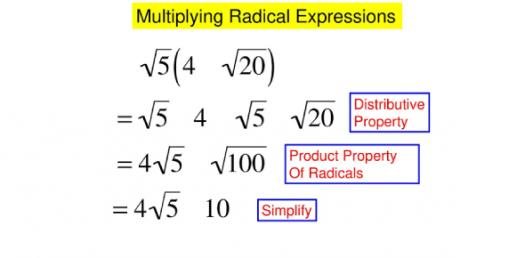# Multiplying Radical Expressions! Math Trivia Questions Quiz

5 QuestionsSettingsThe math trivia questions quiz below is designed to help you solve math problems by multiplying radical expressions! If these problems have been a little hard for you to solve, you are in luck as you will get a chance to have some practice on solving them with each question you tackle. Do give it a shot and get to refresh your understanding!

Related Topics
• 1.
• A.
• B.
• C.
• D.

• 2.
Multiply the following radicals. Simplify if possible.
• A.
• B.
• C.
• D.

• 3.
Multiply the following radicals. Simplify if possible.
• A.
• B.
• C.
• D.

• 4.
Multiply the following radicals. Simplify if possible.
• A.
• B.
• C.
• D.